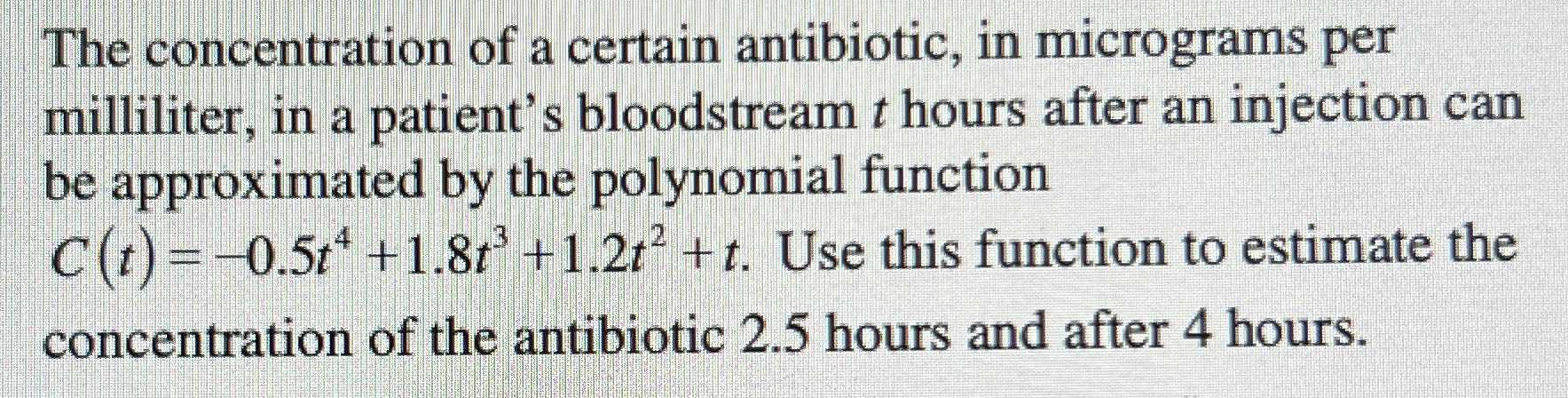### Still have math questions?

Algebra
QuestionThe concentration of a certain antibiotic, in micrograms per milliliter, in a patient's bloodstream t hours after an injection can be approximated by the polynomial function

$$C ( t ) = - 0.5 t ^ { 4 } + 1.8 t ^ { 3 } + 1.2 t ^ { 2 } + t$$ . Use this function to estimate the concentration of the antibiotic $$2.5$$ hours and after $$4$$ hours.

$$- 0.5( 2.5) ^ { 4} + 1.8( 2.5) ^ { 3} + 1.2( 2.5) ^ { 2} + 2.5\\ = 18.59s\\ forx= 4hours\\ - 0.5( 4) ^ { 4} + 1.8( 4) ^ { 3} + 1.2( 4) ^ { 2} + 4\\ = 10.4s$$